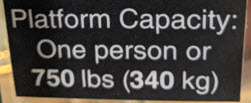B A R E L Y B A D  W E B  S I T E
 Weight
 I don't know why I didn't notice till today (January 28, 2021) this odd sign on a special elevator at one my dentists' offices, which I've been to several times, which I've photographed for you.  The sign plainly implies that one person weighs 750 pounds.At just under 5 feet 11 inches I should weigh, according to the Body Mass Index (BMI), no more than about 176 pounds, whereas in fact I weighed 204 as of this morning, which means I am 16% overweight. (The BMI is calculated by the formula BMI = m / h2, where m is the mass in kilograms and h is the height in meters.  At 204 pounds my mass in kilograms is 204 times a skosh more than 0.453592, which we'll say is 95.528.  At 70.5 inches my height in meters is 70.5 times exactly 0.0254, which is 1.7907.  Plugging the admittedly rounded metric equivalents into the formula, my BMI is 95.5 / 1.82, which is 29.8.  At my height the tipping point is 80 kilograms, which means I need to lose over 15 kilos.  Sheesh.) But according to this sign, at least as I interpret it, one person may weigh as much as 750 pounds.  Do you think a 750-pound human being would come anywhere close to fitting in a typical dentist's chair? If that person was as tall as I am that person would be well over 300% too heavy or that person would be well over 18 feet tall. Who wrote and approved this sign?  Are those people saying, "Ignore this sign and all the others herein"?

 B A R E L Y B A D  W E B  S I T E